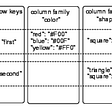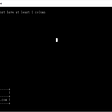# Adding peephole optimization to GCC

`x = (a * b) + (c * d) - e;`
`_1 = a * b_2 = c * d_3 = _1 + _2_4 = _3 - ex = _4`
`x = (a / 2) * 2;  // assume 'a' is unsigned`
`t1 = a / 2;t2 = t1 * 2;x = t2`
`sudo apt install build-essentials flex bisonsudo apt-get build-dep gccmkdir gnu-toolchaincd gnu-toolchaingit clone https://github.com/gcc-mirror/gcccd gcc./contrib/download_prerequisites# Make a *separate* build dir, which is sibling to gcc/cd ..mkdir stage1-buildcd stage1-build../gcc/configure --enable-languages=c,c++ --disable-bootstrap --disable-multilibnohup make -j\$(nproc) >err 2>&1 &`
`double f(double x){    return (__builtin_sin(x) * __builtin_sin(x))           + (__builtin_cos(x) * __builtin_cos(x));}double g(double x){    double t1 = __builtin_sin (x);    double t2 = t1 * t1;    double t3 = __builtin_cos (x);    double t4 = t3 * t3;    return t2 + t4;}`
`;; Function g (g, funcdef_no=1, decl_uid=1909, cgraph_uid=2, symbol_order=1)g (double x){  double t4;  double t3;  double t2;  double t1;  double _6;  complex double sincostmp_8;<bb 2> [local count: 1073741825]:  sincostmp_8 = __builtin_cexpi (x_1(D));  t1_2 = IMAGPART_EXPR <sincostmp_8>;  t2_3 = t1_2 * t1_2;  t3_4 = REALPART_EXPR <sincostmp_8>;  t4_5 = t3_4 * t3_4;  _6 = t2_3 + t4_5;  return _6;}`
`(simplify (plus (mult (SIN @0) (SIN @0))       (mult (COS @0) (COS @0))) (if (flag_unsafe_math_optimizations)  { build_one_cst (TREE_TYPE (@0)); }))`
1. simplify denotes, that the thing is a pattern, which consists of two parts: match, condition and replace.
2. match: The match condition is the expression starting with (plus …). The compiler matches the incoming TREE expression or statements in basic-block against this pattern, and if pattern matching succeeds, it would replace that by the sequence from replacement part.
3. mult denotes the multiplication operator written in lower-case. Built-in functions like sin and cos are denoted by upper case.
4. @0, is a so-called “capture”, which is used to represent an operand, or the “leaf” of the expression. In our case, @0 would be x.
5. The condition (if …) is an actual C condition embedded inside the pattern. This is a kludge, since ideally we want pattern definitions to be only defined with the DSL, but getting down to C becomes necessary when the pattern language isn’t expressive enough, or we neeed to access some other stuff like flag_unsafe_math_optimizations in above case. The transform is gated on the flag to be conservative.
6. Finally coming to replacement part, this can either be a pattern language construct enclosed within (…) or a C expression enclosed within braces { … }. In above example, there’s no construct in pattern language to build tree node, so we “fall back” to C code to construct a tree node of constant 1 with function build_one_cst. The TREE_TYPE macro yields the type of ‘x’, which in our case is double, but could also be other float variants.
`static boolgimple_simplify_273 (gimple_match_op *res_op, gimple_seq *seq,                 tree (*valueize)(tree) ATTRIBUTE_UNUSED,                 const tree ARG_UNUSED (type), tree *ARG_UNUSED (captures), const combined_fn ARG_UNUSED (SIN), const combined_fn ARG_UNUSED (COS)){/* #line 5004 "../../gcc/gcc/match.pd" */  if (flag_unsafe_math_optimizations)    {      gimple_seq *lseq = seq;      if (dump_file && (dump_flags & TDF_FOLDING)) fprintf (dump_file, "Applying pattern match.pd:5005, %s:%d\n", __FILE__, __LINE__);      tree tem;      tem =  build_one_cst (TREE_TYPE (captures));      res_op->set_value (tem);      return true;    }  return false;}`
`f (double x){  double D.1917;  D.1917 = 1.0e+0;   return D.1917;}`
`Visiting statement:t5_6 = t2_3 + t4_5;which is likely CONSTANTMatch-and-simplified t2_3 + t4_5 to 1.0e+0Lattice value changed to CONSTANT 1.0e+0.  Adding SSA edges to worklist.marking stmt to be not simulated againg (double x){  double t5;  double t4;  double t3;  double t2;  double t1;<bb 2> :  t1_2 = __builtin_sin (x_1(D));  t2_3 = t1_2 * t1_2;  t3_4 = __builtin_cos (x_1(D));  t4_5 = t3_4 * t3_4;  return 1.0e+0;`
`g (double x){  <bb 2> [local count: 1073741825]:  return 1.0e+0;}`

--

--

--

## More from Prathamesh Kulkarni

Love podcasts or audiobooks? Learn on the go with our new app.

## CS373 Spring 2021: Brian Wang, Blog #7## Get Hands-On with Decentralized Relational Data## Day 1: CRUD and Table Administration## It’s a (point-)free world## Flask網頁框架學習筆記 — 網頁連接資料庫(含Mysql新增資料庫) #Day 7## .NET: Running Test Cases against a Database [Part 1]## 7 Reasons You Should Enroll in a Coding Boot Camp## Do more with Git’s log command.## Solving the n-queens problem with brute-force search## I tried PyScript and why you shouldn’t? Running Python as a front-end language is not worth it!